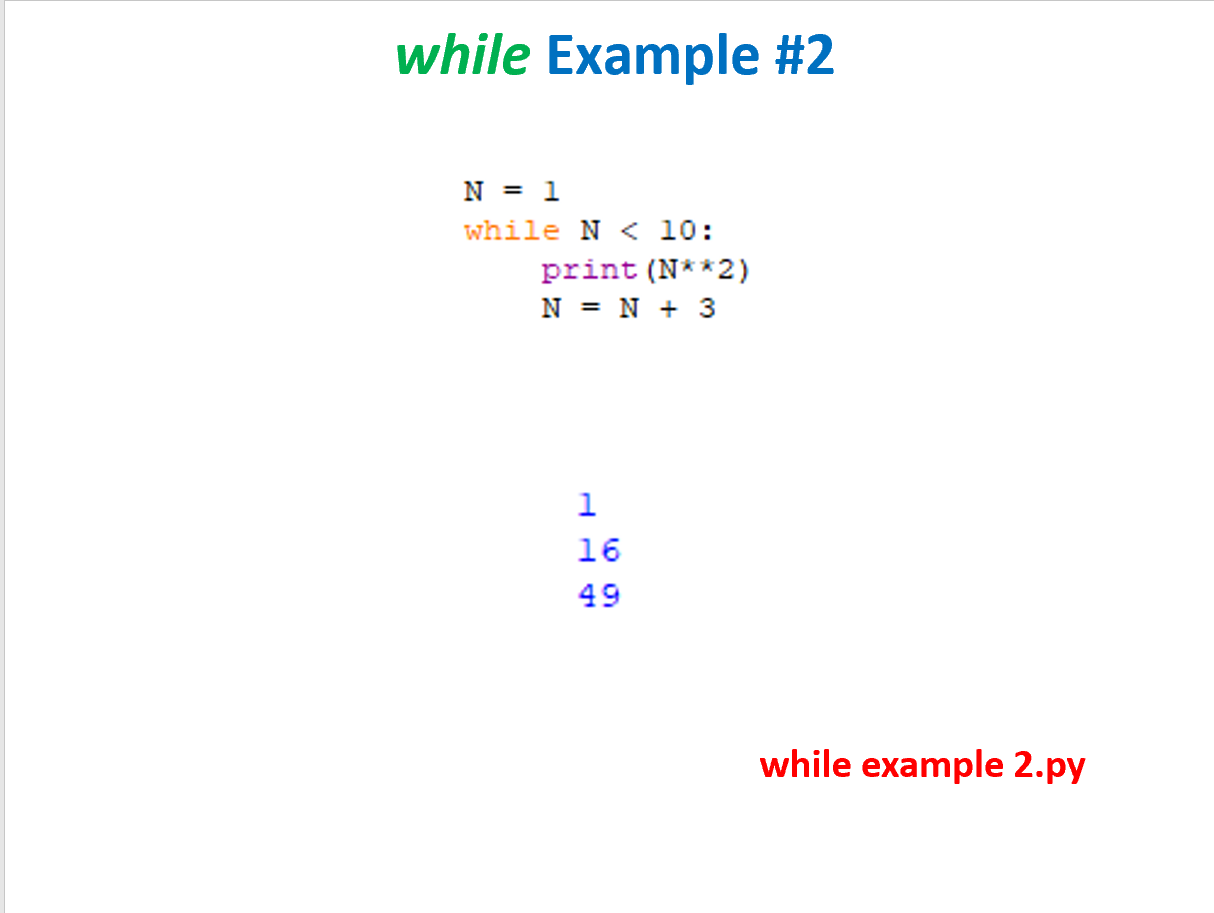# while Example #2N=1while N< 10:print (N* *2)N=N+311649while example 2.py

Question
31 views

I have a Python question and I cannot seem to figure out how to answer this problem exactly (I've attached an example of what the answer should look like-- the picture is to a different problem) :

1. What will be displayed when the following pseudocode is implemented?

• set the variable equal to 0
• repeat the following steps as long as N is less than 4
• display 2 to the power N
• set N equal to N + 1
• display 2 to the power (N + 1)help_outlineImage Transcriptionclosewhile Example #2 N=1 while N< 10: print (N* *2) N=N+3 1 16 49 while example 2.py fullscreen
check_circle

Hey..

To print the power of 2 you have to use a  power function  "pow(2,N) " .

It is inbuild function and you can use it with loading  python   "math"  ...

### Want to see the full answer?

See Solution

#### Want to see this answer and more?

Solutions are written by subject experts who are available 24/7. Questions are typically answered within 1 hour.*

See Solution
*Response times may vary by subject and question.
Tagged in